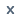Products

• KLQ6109Q

L × W × H : 10490×2500×3590

Seats : 43+1+1, 45+1+1

• KLQ6109T

L × W × H : 10490×2500×3590

Seats : 47/45+1

• KLQ6119T

L × W × H : 10740×2500×3400

Seats : 63/45+1/55+1+1

• KLQ6115H

L × W × H : 10740×2500×3610

Seats : 51/39+1+1, 43+1+1, 47+1+1

• KLQ6112HQ

L × W × H : 10990×2500×3610

Seats : 51/49+1+1, 47+1+1, 45+1+1

• Highway-KLQ6113DFB

L × W × H : 11490×2500×3500

Seats : 51+1

• KLQ6119Q

L × W × H : 11490×2500×3590

Seats : 47+1+1

• Dreamscape-KLQ6122B

L × W × H : 12000×2550×3800

Seats : 59/49+1+1, 51+1+1, 53+1+1

• Utour-KLQ6123K/KLQ6128K

L × W × H : 12000×2550×3820

Seats : 47/45+1+1, 51+1+1

• KLQ6125Q

L × W × H : 12000×2500×3600

Seats : 51+1+1

• KLQ6125A

L × W × H : 12000×2550×3800

Seats : 55/45+1+1

• KLQ6129KA

L × W × H : 12000×2500×3710/3500

Seats : 55/53+1+1, 51+1+1

Previous   1  2  3   4   5  Next Down 5 Page Last75 Records 2/7 Page